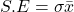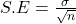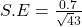## Suppose that a study of elementary school students reports that the mean age at which children begin reading is 5.5 years with a standard de

Question

Suppose that a study of elementary school students reports that the mean age at which children begin reading is 5.5 years with a standard deviation of 0.7 years. Step 2 of 2 : If a sampling distribution is created using samples of the ages at which 43 children begin reading, what would be the standard deviation of the sampling distribution of sample means

in progress 0
6 months 2021-07-17T11:50:44+00:00 1 Answers 12 views 0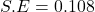Step-by-step explanation:

From the question we are told that:

Mean age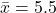standard deviation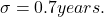Sample size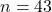Generally the equation for Standard error is mathematically given by